# 量子力学中的波函数与新概念物质波Wave Functions in Quantum Mechanics and the New Concept of Matter Waves

• 全文下载: PDF(959KB)    PP.206-226   DOI: 10.12677/MP.2019.95023
• 下载量: 272  浏览量: 1,399

Because the experiment proves that the Copenhagen about “wave function does not represent the real matter waves but subjective in the sense of probability wave” point of view is wrong, the wave function is objective in description of matter waves, thus to reveal the form of the objective exist-ence of matter waves and properties, this article embarks from the concept of DE Broglie wave guide, by analogy with Einstein’s theory of light quantum theory, puts forward that any column objective existence of matter wave energy is quantized, the particle is accompanied with the as-sumption, the quantized energy existing in the matter waves on the basis of analysis found that any column in the form of matter waves are waves, thus the new concept of wave of matter must and can only be described by wave functions in quantum mechanics. After analyzing and reasoning, the following conclusions are obtained: the matter wave of any particle follows the superposition principle of matter wave; Schrodinger equation in quantum mechanics can be established from the concept of matter wave. The statistical interpretation of wave function can be understood by means of the concept of matter wave, and Schrodinger’s cat paradox and EPR paradox (or quantum entanglement) can also be solved on the basis of the new concept of matter wave. The uncertainty relation in quantum mechanics can be explained by the concept of matter wave. The concept of matter wave is falsifiable, and its existence has been verified by physical experiments, so it is a scientific concept.

1. 引言

2. 物质波及其能量与动量

${V}_{g}=\underset{\Delta \omega \to 0,\Delta k\to 0}{\mathrm{lim}}\frac{\Delta \omega }{\Delta k}=\text{d}\omega /\text{d}k=V$ (1)

$E=\frac{m{C}^{2}}{\sqrt{1-{V}^{2}/{C}^{2}}}$ (2)

${E}_{k}=m{C}^{2}-{m}_{0}{C}^{2}$ (3)

$p=\frac{mV}{\sqrt{1-{V}^{2}/{C}^{2}}}$ (4)

${E}^{2}={p}^{2}{C}^{2}+{m}_{0}^{2}{C}^{4}$ (5)

3. 物质波的存在形式及其描述方法

3.1. 物质波的存在形式

3.2. 物质波的描述方法

$\Psi \left(r,t\right)={\Psi }_{0}{\text{e}}^{i\left(k\cdot r-\omega t\right)}$ (6)

$\Psi \left(r,t\right)={\Psi }_{0}{\text{e}}^{\frac{i}{\hslash }\left(p\cdot r-Et\right)}$ (7)

 (8)

$c\left(p,t\right)=\frac{1}{{\left(2\text{π}\hslash \right)}^{3/2}}\underset{-\infty }{\overset{+\infty }{\int \text{ }\int \text{ }\int }}\Psi \left(r,t\right){\text{e}}^{-\frac{i}{\hslash }p\cdot r}\text{d}x\text{d}y\text{d}z$ (9)

4. 物质波须遵循的规律

4.1. 物质波的叠加原理

$\Psi ={C}_{0}{\Psi }_{0}+{C}_{1}{\Psi }_{1}+\cdots +{C}_{n}{\Psi }_{n}={\sum }_{i=0}^{i=n}{C}_{i}{\Psi }_{i}$ (10)

$\Psi ={C}_{0}{\Psi }_{0}+{C}_{1}{\Psi }_{1}+\cdots +{C}_{n}{\Psi }_{n}=\underset{i=0}{\overset{i=n}{\sum }}{C}_{i}{\Psi }_{i}$ (11)

4.2. 物质波的干涉及衍射

4.2.1 . 物质波的双缝干涉Figure 1. Experimental diagram of electronic double-slit interference

${\Psi }_{0}={\Psi }_{1}+{\Psi }_{2}={\Psi }_{A}{\text{e}}^{-i\omega t}\left(1+{\text{e}}^{i2\pi l\mathrm{sin}\theta /\lambda }\right)$ (12)

${|{\Psi }_{0}|}^{2}={|{\Psi }_{1}+{\Psi }_{2}|}^{2}=4{|{\Psi }_{A}|}^{2}{\mathrm{cos}}^{2}\left(\frac{\pi l\mathrm{sin}\theta }{\lambda }\right)$ (13)

$\pi l\mathrm{sin}\theta /\lambda$ 等于  时， ${|{\Psi }_{0}|}^{2}=4{|{\Psi }_{A}|}^{2}$ 为最大值，因而有

$\mathrm{sin}\theta =n\lambda /l$ (14)

$\mathrm{sin}\theta =\left(2n-1\right)\lambda /2l$ (15)

4.2.2. 物质波的衍射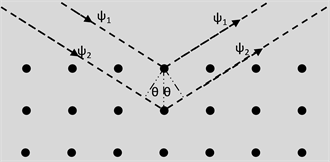Figure 2. Experimental diagram of electron nickel crystal diffraction

5. 物质波的波动方程

$\frac{\partial \Psi }{\partial t}=-\frac{i}{\hslash }E\Psi$ (16)

$\frac{{\partial }^{2}\Psi }{\partial {x}^{2}}+\frac{{\partial }^{2}\Psi }{\partial {y}^{2}}+\frac{{\partial }^{2}\Psi }{\partial {z}^{2}}=-\frac{{p}^{2}}{{\hslash }^{2}}\Psi$ (17)

$E=\frac{{p}^{2}}{2m}$ (18)

$i\hslash \frac{\partial \Psi }{\partial t}=-\frac{{\hslash }^{2}}{2m}\left(\frac{{\partial }^{2}\Psi }{\partial {x}^{2}}+\frac{{\partial }^{2}\Psi }{\partial {y}^{2}}+\frac{{\partial }^{2}\Psi }{\partial {z}^{2}}\right)=-\frac{{\hslash }^{2}}{2m}{\nabla }^{2}\Psi$ (19)

$i\hslash \frac{\partial }{\partial t}\Psi =E\Psi$ (20)

$-i\hslash \left(i\frac{\partial }{\partial x}\Psi +j\frac{\partial }{\partial y}\Psi +k\frac{\partial }{\partial z}\Psi \right)=\left(i{p}_{x}+j{p}_{y}+k{p}_{z}\right)\Psi =p\Psi$ (21)

$E=\frac{{p}^{2}}{2m}+U\left(r\right)$ (22)

$i\hslash \frac{\partial \Psi }{\partial t}=-\frac{{\hslash }^{2}}{2m}{\nabla }^{2}\Psi +U\left(r\right)\Psi$ (23)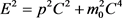(5a)

$-{\hslash }^{2}\frac{{\partial }^{2}\Psi }{\partial {t}^{2}}=-{\hslash }^{2}{C}^{2}{\nabla }^{2}\Psi +{m}_{0}^{2}{C}^{4}\Psi$ (24)

$E=\sqrt{{P}^{2}{C}^{2}+{m}_{0}^{2}{C}^{4}}$ (25)

$i\hslash \frac{\partial \Psi }{\partial t}=\left(-i\hslash C\alpha \cdot \nabla +\beta {m}_{0}{C}^{2}\right)\Psi$ (26)

${\alpha }_{x}=\left[\begin{array}{cc}\begin{array}{cc}0& 0\\ 0& 0\end{array}& \begin{array}{cc}0& 1\\ 1& 0\end{array}\\ \begin{array}{cc}0& 1\\ 1& 0\end{array}& \begin{array}{cc}0& 0\\ 0& 0\end{array}\end{array}\right]$ , ${\alpha }_{y}=\left[\begin{array}{cc}\begin{array}{cc}0& 0\\ 0& 0\end{array}& \begin{array}{cc}0& -i\\ i& 0\end{array}\\ \begin{array}{cc}0& -i\\ i& 0\end{array}& \begin{array}{cc}0& 0\\ 0& 0\end{array}\end{array}\right]$ , ${\alpha }_{z}=\left[\begin{array}{cc}\begin{array}{cc}0& 0\\ 0& 0\end{array}& \begin{array}{cc}1& 0\\ 0& -1\end{array}\\ \begin{array}{cc}1& 0\\ 0& -1\end{array}& \begin{array}{cc}0& 0\\ 0& 0\end{array}\end{array}\right]$ , $\beta =\left[\begin{array}{cc}\begin{array}{cc}1& 0\\ 0& 1\end{array}& \begin{array}{cc}1& 0\\ 0& 0\end{array}\\ \begin{array}{cc}0& 0\\ 0& 0\end{array}& \begin{array}{cc}-1& 0\\ 0& -1\end{array}\end{array}\right]$

6. 粒子的运动规律

6.1. 粒子的力学量的概率分布

$\text{d}W\left(x,y,z,t\right)=K{|\Psi \left(x,y,z,t\right)|}^{2}\text{d}\tau$ (27)

$w\left(x,y,z,t\right)=\frac{\text{d}W\left(x,y,z,t\right)}{\text{d}\tau }=K{|\Psi \left(x,y,z,t\right)|}^{2}$ (28)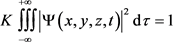(29)

$K=\frac{1}{{\int \text{ }\int \text{ }\int }_{-\infty }^{+\infty }{|\Psi \left(x,y,z,t\right)|}^{2}\text{d}\tau }$ (30)

$\underset{-\infty }{\overset{+\infty }{\int \text{ }\int \text{ }\int }}{|\psi \left(x,y,z,t\right)|}^{2}\text{d}\tau =1$ (31)

6.2. 粒子的力学量的测量与薛定谔猫悖论及EPR悖论

6.2.1. 薛定谔猫悖论分析

6.2.2. EPR悖论分析

1951年，D·玻姆提出了玻姆版本的EPR悖论，又称为“EPRB悖论”，该版本悖论所涉及的实验是助施特恩–格拉赫仪器测量粒子沿z轴方向的离散自旋。假设一个0自旋的中性π介子衰变成一个电子与一个正电子，这两个衰变产物各自朝相反方向运动。当电子运动至空间坐标A时该点上的观察者a将观测到电子沿z轴方向的自旋；而当正电子运动到空间坐标B时该点上的观察者b将观测到正电子沿z轴方向的自旋。这两个纠缠粒子共同形成零自旋单态，是两个直积态的叠加。若不做测量，则无法知道这两个粒子中任何一个粒子沿z轴的自旋，并且根据哥本哈根诠释，这个变量并不存在。这个单态具有旋转不变性，对于任意取向的参考轴，它保持同样的性质。这个单态的两个粒子相互反关联，测量沿着z轴的自旋，假若电子自旋为+1/2，则正电子自旋为−1/2，假若电子自旋为−1/2，则正电子自旋为+1/2。量子力学不能预测到底是哪一组数值，但可以预测获得任何一组数值的概率为50%，对这种情况，物理学家称电子与正电子处于量子纠缠态。

$|\text{A},\text{B}〉=\frac{1}{\sqrt{2}}\left[{|{↓}_{\text{z}}〉}_{\text{B}}{|{↑}_{\text{z}}〉}_{\text{A}}-{|{↑}_{\text{z}}〉}_{\text{B}}{|{↓}_{\text{z}}〉}_{\text{A}}\right]$ (32)

6.3. 粒子的非对易力学量之间的测不准关系

$\Delta x\cdot \Delta {p}_{x}\ge \frac{\hslash }{2},\text{\hspace{0.17em}}\text{\hspace{0.17em}}\Delta y\cdot \Delta {p}_{y}\ge \frac{\hslash }{2},\text{\hspace{0.17em}}\text{\hspace{0.17em}}\Delta z\cdot \Delta {p}_{z}\ge \frac{\hslash }{2}$ (33)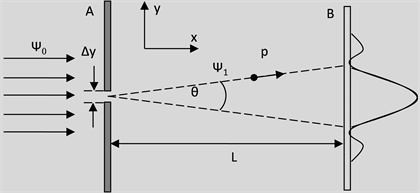Figure 3. Experimental diagram of single slit diffraction of electrons

$\Delta {x}^{2}=\frac{{\int }_{-\infty }^{+\infty }{\left(x-{x}_{0}\right)}^{2}{|\psi \left(x\right)|}^{2}\text{d}x}{{\int }_{-\infty }^{+\infty }{|\psi \left(x\right)|}^{2}\text{d}x},\text{\hspace{0.17em}}\text{\hspace{0.17em}}\Delta {y}^{2}=\frac{{\int }_{-\infty }^{+\infty }{\left(y-{y}_{0}\right)}^{2}{|\psi \left(y\right)|}^{2}\text{d}y}{{\int }_{-\infty }^{+\infty }{|\psi \left(y\right)|}^{2}\text{d}y},\text{\hspace{0.17em}}\text{\hspace{0.17em}}\Delta {x}^{2}=\frac{{\int }_{-\infty }^{+\infty }{\left(z-{z}_{0}\right)}^{2}{|\psi \left(z\right)|}^{2}\text{d}z}{{\int }_{-\infty }^{+\infty }{|\psi \left(z\right)|}^{2}\text{d}z}$ (34)

$\begin{array}{l}\Delta {p}_{x}^{2}=\frac{{\int }_{-\infty }^{+\infty }{\left({p}_{x}-{p}_{x}^{0}\right)}^{2}{|\psi \left({p}_{x}\right)|}^{2}\text{d}{p}_{x}}{{\int }_{-\infty }^{+\infty }{|\psi \left({p}_{x}\right)|}^{2}\text{d}{p}_{x}},\text{\hspace{0.17em}}\text{\hspace{0.17em}}\Delta {p}_{y}^{2}=\frac{{\int }_{-\infty }^{+\infty }{\left({p}_{y}-{p}_{y}^{0}\right)}^{2}{|\psi \left({p}_{y}\right)|}^{2}\text{d}{p}_{y}}{{\int }_{-\infty }^{+\infty }{|\psi \left({p}_{y}\right)|}^{2}\text{d}{p}_{y}},\text{\hspace{0.17em}}\text{\hspace{0.17em}}\\ \Delta {p}_{z}^{2}=\frac{{\int }_{-\infty }^{+\infty }{\left({p}_{z}-{p}_{z}^{0}\right)}^{2}{|\psi \left({p}_{z}\right)|}^{2}\text{d}{p}_{z}}{{\int }_{-\infty }^{+\infty }{|\psi \left({p}_{z}\right)|}^{2}\text{d}{p}_{z}}\end{array}$ (35)

$\Delta x\cdot \Delta {p}_{x}\ge \hslash /2,\text{\hspace{0.17em}}\text{\hspace{0.17em}}\Delta y\cdot \Delta {p}_{y}\ge \hslash /2,\text{\hspace{0.17em}}\text{\hspace{0.17em}}\Delta z\cdot \Delta {p}_{z}\ge \hslash /2$ (33a)

$\Delta t\cdot \Delta E\ge \hslash /2$ (36)

$\stackrel{¯}{{\left(\Delta {L}_{x}\right)}^{2}}\stackrel{¯}{{\left(\Delta {L}_{y}\right)}^{2}}\ge \frac{{m}^{2}{\hslash }^{2}}{4}$ (37)

7. 物质波存在的实验验证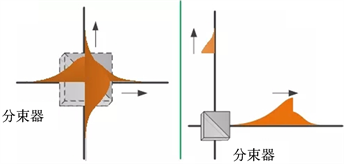(a) (b)

Figure 4. (a) Indicates that when the two-way wave function meets at the interferometer exit, the beam splitter is placed; (b) Indicates changes in the spatial morphology of a single photon due to interference by a subwave function caused by a beam splitterFigure 5. An experimental diagram of the existence of the object described by the photon wave function

8. 结论

1) 任何粒子的物质波都必须遵循物质波的叠加原理，而这正是所谓粒子的态的叠加原理的本质，并且各种粒子的双缝干涉及单缝衍射现象都是物质波的叠加原理的具体体现，同时这些现象均可在新概念物质波基础上获得真正意义上的理解；

2) 量子力学中的薛定谔方程、克莱因–高登方程及狄拉克方程等是在不同速度条件下物质波的波动方程的具体形式，这些方程均可由新概念物质波出发得以建立，从而可重建(而非简单的重复)量子力学；

3) 波函数统计解释的本质为用于描述粒子的态的波函数其描述的对象就是客观存在且其能量具有量子化特征的物质波，因而完全可以借助新概念物质波得以理解，并且薛定谔猫悖论及EPR悖论(或量子纠缠)也可以在新的物质波概念基础上得以圆满解决；

4) 粒子的任意两个具有非对易关系的力学量之间都存在的测不准关系是波函数所描述的物质波的叠加原理的具体表现形式，因而完全可以借助新概念物质波进行诠释；

5) 新概念物质波具有可证伪性，其存在已获得有关物理学实验的证实，因而是科学的概念；

6) 哥本哈根学派关于“波函数不代表真实的物质波而只是主观意义上的概率波”的观点是错误的。

  李宏芳. 量子理论的观念之争和认识论发展[M]. 北京: 科学出版社, 2015: 19.  中国科学物理辑中国科学杂志社. 相遇延迟实验和量子力学波函数的实在性本质[EB/OL]. https://mp.weixin.qq.com, 2018-01-09.  德布罗意. 德布罗意文选[M]. 北京: 北京大学出版社, 2016: 9, 111, 140, 149.  张永德. 量子力学[M]. 第4版. 北京: 科学出版社, 2017: 7, 323.  朱栋培. 量子力学基础[M]. 合肥: 中国科学技术大学出版社, 2016: 9.  顾樵. 量子力学I[M]. 北京: 科学出版社, 2018: 50.  张永德. 量子力学[M]. 第4版. 北京: 科学出版社, 2017: 7, 13.  朱栋培. 量子力学基础[M]. 合肥: 中国科学技术大学出版社, 2016: 137-139.  朱栋培. 量子力学基础[M]. 合肥: 中国科学技术大学出版社, 2016: 10.  Long, G.L., Qin, W., Yang, Z. and Li, J.-L. (2018) Realistic Interpretation of Quantum Mechanics and Encounter-Delayed-Choice Experiment. Science China Physics, Mechanics & Astronomy, 61, Article ID: 030311. https://doi.org/10.1007/s11433-018-9162-4